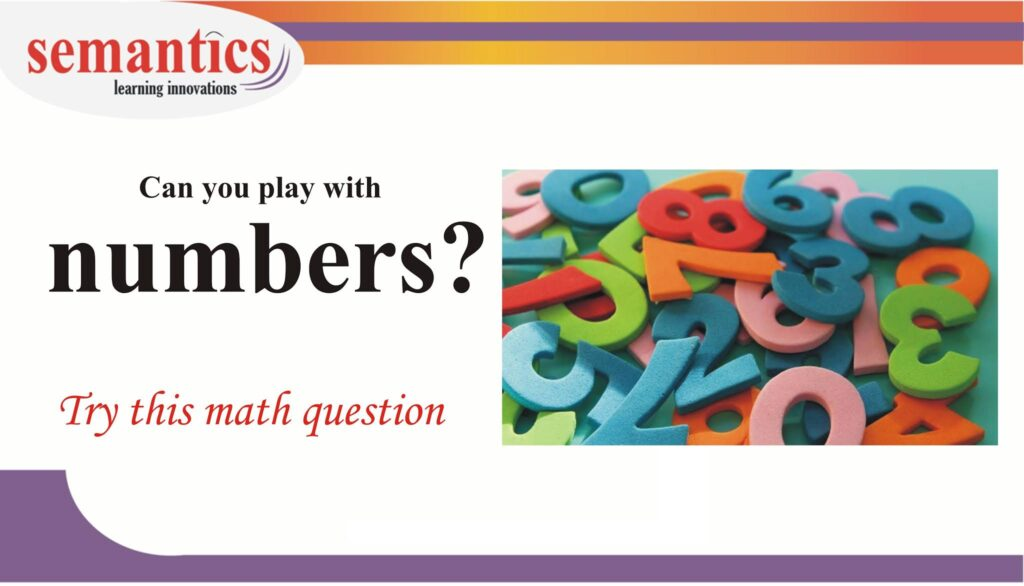# Math Reasoning Skill – playing with numbersSome question, in the GMAT /GRE,  test your ability to reason with numbers.
That too, in 30 to 45 seconds.

So while preparing for an exam of this caliber,focus on

• learning concepts
• reasoning skills.

## One of the math reasoning skills, you must focus on, is Playing with numbers.

Focus on:

Factors and multiples · Prime and composite numbers · Tests for divisibility · Common factors and common multiples · Prime factorization…..

Try this sum

In an auditorium, 360 chairs are to be set up in a rectangular arrangement with x rows of exactly y chairs each. If the only other restriction is that 10 < x < 25, how many different rectangular arrangements are possible?

A. 4        B. 5         C.6        D.8         E.9

This sum is based on number properties. There are two methods to solve this problem.

Method 1 – the conventional wayYou should know the concept of prime factorization.

As per the question

X rows x Y chairs = 360

The product of two integers = 360.

Now Let’s find the prime factorization of 360

360 = 2 x 2 x 2 x 3 x 3 x 5

As per the condition given in the question The value of x should lie between 10 and 25. The value of X should be a combination of the prime factors of 360

360 = 2 x 2 x 2 x 3 x 3 x 5

Pick few of the above numbers and find the product ( the product should lie between 10 and 25)

2 x 2 x 3 = 12

3 x 5 = 15. and so on…

Hence X can have values 12, 15,18, 20,24

The pairs are

x = 12 & y = 30
x = 15 & y = 24
x = 18 & y = 20
x = 20 & y = 18
x = 24 & y = 15

There are five such possibilities

Method 2 – Playing with numbers..Let us say you didn’t know the concept of prime factorization, then you can play with numbers and arrive at the answer.

X is a number between 10 and 25.  The Possible values of x are

11 12 13 14 15 16 17 18 19 20 21 22 23 24

(one of these numbers) x (a unknown number) = 360

Did you notice that there are prime numbers in the middle.( 11,13….23)

None of the prime numbers divide 360 – 13,17,19, 23

Rule out the primes. Whats left………….

12 14 15 16 18 20 21 22 24

(one of these numbers) x (a new number) = 360

This means 360 is perfectly divisible by either of these two numbers.

Take one number at a time

360 /12 .. its divisible … keep it..

360/14 = 360/(7×2) is not an integer ….360 is not divisible by 7.. rule it out

360/16 = 360/(4×4) is not an integer… 360 is not divisible by 4 twice.. rule it out

360/21 = 360/(7×3) is not an integer….360 is not divisible by 7.. rule it out

360/22 = 360/(2×11) is not an integer…360 is not divisible by 11.. rule it out

Whats left.. 12 15 18 20 24

There are five such possibilities## What next? Master this skills with a small test. click here

Hope you understood both the methods. Even if you are out of touch with math ..

With logic you can ace this section…

If you need any help math help in your  prep… Contact me…

My contact details are here: